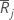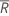# Methods and formulas for Kruskal-Wallis Test

Select the method or formula of your choice.

## Average rank

Minitab calculates the average ranks as follows:
1. Ranks the combined samples, assigns the smallest observation a rank of 1, the second smallest observation a rank of 2, and so on.
2. If two or more observations are tied, Minitab assigns the average rank to both observations.
3. Calculates the average of the ranks of each sample.

Minitab displays the values for each group under Ave Rank in the output.

## Z-value

### Formula

Minitab calculates the z-value for each group as follows:

### Notation

TermDescriptionaverage rank for group javerage rank for all observations
Nnumber of observations
njnumber of observations for the jth group

## Ranking tied values

Tied values occur when two or more observations are equal. If your data has tied values, Minitab ranks the data as follows:
1. Sort the observations in ascending order.
2. Assign ranks to each observation as if there were no ties.
3. For a tied set, take the average of the corresponding ranks and assign this value as the new rank to each tied value in that set.

### Example

A sample has 9 observations: 2.4, 5.3, 2.4, 4.0, 1.2, 3.6, 4.0, 4.3, and 4.0

Observation Rank (assuming no ties) Rank
1.2 1 1
2.4 2 2.5
2.4 3 2.5
3.6 4 4
4.0 5 6
4.0 6 6
4.0 7 6
4.3 8 8
5.3 9 9

The following information is also used when calculating the test statistics:
• The number of sets of ties = 2
• The number of tied values in the first set = 2
• The number of tied values in the second set = 3

## H

### Formula

Under the null hypothesis, the chi-square distribution with k – 1 degrees of freedom approximates the distribution of H. The approximation is reasonably accurate when no group has fewer than five observations. A higher H value provides stronger evidence for the null hypothesis that the difference between some of the medians is statistically significant.

Some authors, such as Lehmann (1975)1, suggest adjusting H when the data have ties. Minitab displays H(adj) when the data have ties.

Under the null hypothesis, the chi-square distribution with k – 1 degrees of freedom approximates the distribution of H and H(adj).

P-value = 1 – CDF (χ2H, df)

P-value = 1 – CDF (χ2H(adj), df)

For small samples, Minitab recommends that you use exact tables. For more details, see Hollander and Wolfe (1973)2.

### Notation

TermDescription
nj number of observations in group j
Ntotal sample sizeaverage of the ranks in group javerage of all of the ranks
tinumber of tied values in the ith set of ties
1 E.L. Lehmann (1975). Nonparametrics: Statistical Methods Based on Ranks, Holden-Day.
2 M. Hollander and D.A. Wolfe (1973). Nonparametric Statistical Methods, John Wiley & Sons, Inc.
By using this site you agree to the use of cookies for analytics and personalized content.  Read our policy